# GSEB Solutions Class 9 Maths Chapter 4 Linear Equations in Two Variables Ex 4.3

Gujarat Board GSEB Solutions Class 9 Maths Chapter 4 Linear Equations in Two Variables Ex 4.3 Textbook Questions and Answers.

## Gujarat Board Textbook Solutions Class 9 Maths Chapter 4 Linear Equations in Two Variables Ex 4.3

Question 1.
Draw the graph of each of the following linear equations in two variaNes.
(i) x + y = 4
(ii) x – y = 2
(iii) y = 3x
(iv) 3 = 2x + y
Solution:
(i) x + y = 4
y = 4 – x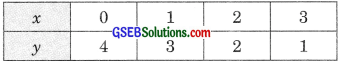Now plot the points (0, 4), (1, 3), (2, 2) and (3, 1) on the graph paper and join them by using a ruler to get the line which is the graph of the equation x + y = 4.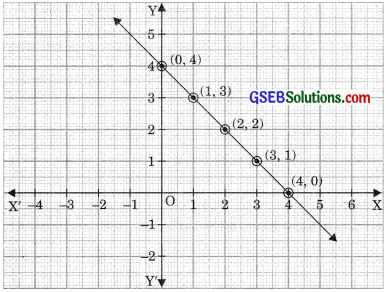img

(ii) x – y = 2
x = y + 2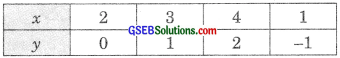Now plot the points (2, 0), (3, 1), (4, 2) and (1, -1) on the graph paper and join these points by using a ruler to get the line which is the graph of the equation x – y = 2.(iii) y = 3xNow plot the points (2, 0), (3, 1), (4, 2) and (1, -1) on the graph paper and join these points by the ruler to get the line which is the graph of the equation x-y = 2.(iv) 3 = 2x +y
y = 3 – 2xNow plot the points (0, 3), (1, 1), (2, -) and (-1, 5) on the graph paper and join these points by the ruler to get the line which is the graph of the equation 3 = 2x + y.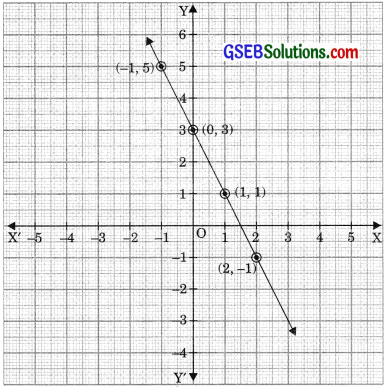Question 2.
Give the equations of two lines passing through (2, 14). How many more such lines are there, and why?
Solution:
Equation of two lines which passes through (2, 14) are x + y = 16
and 2x + y = 18
There are infinitely many lines which can pass through the given point. Because there are many lines which can pass through a point.Question 3.
If the point (3, 4) lies on the graph of the equation 3y = ax + 7, find the value of a.
Solution:
If the point (3, 4) lies on the graph of the equation 3y = ax + 7, then
3y = ax + 7
⇒ 3 x 4 = a x 3 + 7
⇒ 12 = 3a + 7
⇒ 3a = 5
⇒ a = $$\frac {5}{3}$$

Question 4.
The taxi fare in a city is as follows for the first kilometre, the fare is 8 and for the subsequent distance, it is 5 per km. Taking the distance covered as x km and total fare as y. Write a linear equation for this information and draw its graph.
Solution:
Total distance covered = x km
Total fare = ₹ y
Fare for the first km = ₹ 8
Subsequent distance = (x – 1) km
Fare for subsequent distance = ₹ 5(x – 1)
According to the problem,
y = 8 + 5(x – 1) = 8 + 5x – 5
⇒ y = 3 + 5x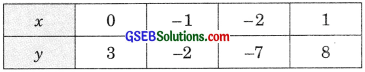Question 5.
From the choice given below, choose the equation whose graphs are given in figure 1 and fig. 2 for fig. 1 for fig. 2
(i) y = x   (i) y = x+2
(ii) x + y = 0 (ii) y = x – 2
(iii) y = 2x  (iii) y = -x + 2
(iv) 2 + 3y = 7  (iv) x + 2y = 6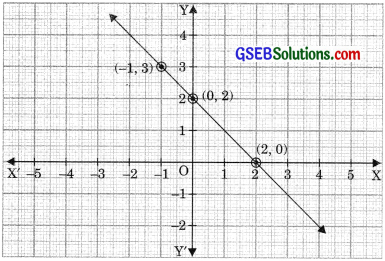Solution:
For fig. (i) the correct equation is (ii) x + y = O as (-1, 1), (0, 0) and (1, -1) satisfy the equation x + y = 0. For fig. (2) the correct equation is (iii) y = -x + 2 as (-1, 3), (0, 2) and (2, 0) satisfy the equation y = -x + 2Question 6.
If the work done by a body on application of a constant force is directly proportional to the distance travelled by the body, express this in the form of an equation in two variables and draw the graph of the same by taking the constant force as 5 units. Also read from the graph the work done when the distance travelled by the body is (i) 2 units (ii) 0 unit
Solution:
Let distance travelled by the body be x units and the work done by the constant force be y units. Given constant force is 5 units.
Work done = Force x Displacement
y = 5xNow plot the points (0, 0), (1, 5 and (-1, -5) on the graph paper and join these points by a ruler to get a line which is the graph of the equation y = 5x.(i) 2 units Through the point A(2, 0) draw a line parallel toy-axis which intersects the graph of the equation y = 5x at B. And through the point B draw a line parallel to x-axis which intersects OY at the point C(0, 10). Work done when the distance travelled by the body is 2 units is 10 units.

(ii) From the graph it is clear that x = 0 corresponds to y = 0. Hence, when distance travelled is 0 units (x = 0), then work done, y = 0 units.

Question 7.
Yamini and Fatima, two students of class IX of a school, together contributed loo towards the Prime minister’s relief fund to help the earthquake victims. Write a linear equation which satisfies this data. (You take their contributions as x and y). Draw the graph of the same.
Solution:
Let the contribution of Yamini and Fatima towards Prime minister relief fund to hell) earthquake victims be x and y respectively.
Then according to the problem
x + y = 100
y = 100 – xNow plot the points (0, 100), (25, 75), (50, 50) (75, 25) and (100, 0) on the graph paper and join these points by the ruler to get line which is the graph of line x + y = 100.Question 8.
In countries like USA and Canada, the temperature is measured in Fahrenheit. Whereas in countries like India it is measured in Celsius. Here is a linear equation that converts Fahrenheit to Celsius.
F = ($$\frac {1}{2}$$) C + 32
(i) Draw the graph of the linear equation above using Celsius for x-axis and Fahrenheit for the y-axis.
(ii) If the temperature is 30°C, what is the temperature in Fahrenheit?
(iii) If the temperature is 95°F, what is the temperature in Celsius?
(iv) If the temperature is 0°C, what is the temperature in Fahrenheit and if the temperature is 0°F. What is the temperature in Celsius?
(v) Is there a temperature which is numerically the same in both Fahrenheit and Celsius? If yes, find it.
Solution:
(i) F = $$\frac {1}{2}$$ C + 32Now plot these points i.e., (0, 32), (5, 41), (10, 50) and (15, 59) on the graph paper and join these points by ruler to get line which is the graph of the equation
F = $$\frac {1}{2}$$ C + 32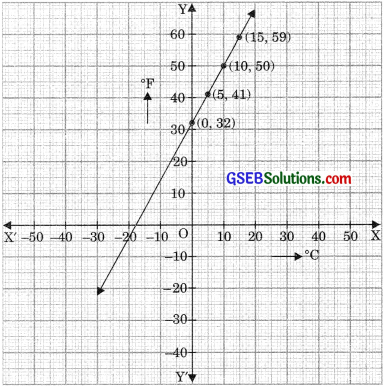(ii) F = $$\frac {1}{2}$$ C + 32
When C = 30, then
F = $$\frac {9}{5}$$ x 30 + 32
= 9 x 6 + 32 = 54 + 32
⇒ F = 86
Thus, required temperature = 86°F(iii) When F = 95, then
F = $$\frac {9}{5}$$ x C + 32
⇒ 95° – 32 = $$\frac {9}{5}$$ C ⇒ 63 x $$\frac {5}{9}$$ = C
⇒ 7 x 5 = C
⇒ C = 35°
Thus required temperature is 35°C.

(iv) When C = 0, then
F = $$\frac {9}{5}$$ x C + 32 = $$\frac {9}{5}$$ x 0 + 32
F = 32
Thus, the temperature is 32°F.
When F = 0 then
F = $$\frac {9}{5}$$ C + 32
$$\frac {9}{5}$$ C = -32
⇒ C = – 32 x $$\frac {5}{9}$$
C = $$\frac {-160}{9}$$ C
Required temperature is $$\frac {-160}{9}$$ C

(v) Let x be the temperature which would be numerically the same in both Fahrenheit and Celsius scale.
Then, F = $$\frac {9}{5}$$ C + 32
⇒ x = $$\frac {9}{5}$$ x + 32
⇒ – 32 = $$\frac {9}{5}$$ x – x
⇒ – 32 = $$\frac {4}{5}$$ x
x = $$\frac {-32 x 5}{4}$$
x = -40
∴ Required temperature is -40°C = -40°F.